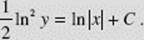﻿ ﻿SOLVING FIRST-ORDER DIFFERENTIAL EQUATIONS ANALYTICALLY - Differential Equations - Calculus AB and Calculus BC

## CHAPTER 9 Differential Equations

### D. SOLVING FIRST-ORDER DIFFERENTIAL EQUATIONS ANALYTICALLY

In the preceding sections we solved differential equations graphically, using slope fields, and numerically, using Euler’s method. Both methods yield approximations. In this section we review how to solve some differential equations exactly.

Separating Variables

A first-order d.e. in x and y is separable if it can be written so that all the terms involving y are on one side and all the terms involving x are on the other.

A differential equation has variables separable if it is of the formThe general solution isEXAMPLE 8

Solve the d.e.given the initial condition y(0) = 2.

SOLUTION: We rewrite the equation as y dy = −x dx. We then integrate, gettingSince y(0) = 2, we get 4 + 0 = C; the particular solution is therefore x2 + y2 = 4. (We need to specify above that y > 0. Why?)

EXAMPLE 9

Ifand t = 0 when s = 1, find s when t = 9.

SOLUTION: We separate variables:then integration yieldsUsing s = 1 and t = 0, we getso C = + 2. ThenWhen t = 9, we find that s1/2 = 9 + 1, so s = 100.

EXAMPLE 10

If (ln y)and y = e when x = 1, find the value of y greater than 1 that corresponds to x = e4.

SOLUTION: Separating, we getWe integrate:Using y = e when x = 1 yieldssoWhen x = e4, we havethus ln2 y = 9 and ln y = 3 (where we chose ln y > 0 because y > 1), so y = e3.

EXAMPLE 11

Find the general solution of the differential equationSOLUTION: We rewriteTaking antiderivatives yields eu = ev + C, or u = ln(ev + c).

﻿